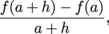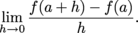Study Guide

## Derivatives as an Instantaneous Rate of Change

Here we'll approach the derivative from a different and more real-lifey direction.

There are two ways to think about speed while driving. The first way is to look at the speedometer, which shows how fast the vehicle is going at that moment. The other way is to take the number of miles driven and divide by how long the drive took. The first way tells the "instantaneous" speed—the speed at that instant. The second way gives average speed over the whole trip.

Although looking at the speedometer may seem like the best way to figure out speed, in order to relate this driving stuff properly to derivatives we need to talk about average speed first.

• ### Average Rate of Change

A rate is a value that expresses how one quantity changes with respect to another quantity. For example, a rate in "miles per hour" expresses the increase in distance with respect to the number of hours we've been driving.

If we drive at a constant rate, the distance we travel is equal to the rate at which we travel multiplied by time:Dividing both sides by time, we have### Sample Problem

If we drive at 50 mph for two hours, the distance we'll travel is 50 mph × 2 hrs = 100 miles.

### Sample Problem

If we drive at a constant speed for 3 hours and travel 180 miles, we must have been driving.

In real life, though, we don't drive at a constant rate. When we start our trip through Shmoopville, we first climb into the car, traveling at a whopping 0 miles per hour. We speed up gradually (hopefully), maybe need to slow down and speed up again for traffic lights, and finally slow down back to a speed of 0 when reaching our destination, The Candy Stand. We can still divide the distance we travel by the time it takes for the trip, but now we'll find our average rate:To calculate the average rate of change of a dependent variable y with respect to the independent variable x on a particular interval, we need to know

• the size of the interval for the independent variable, and

• the change in the dependent variable from the beginning to the end of the interval.

Depending on the problem, we may also need to know

• the units of the independent and dependent variables.

Then we can findThe average rate of change of y with respect to x is the slope of the secant line between the starting and ending points of the interval:Relating this to the more math-y approach, think of the dependent variable as a function f of the independent variable x. Let h be the size of the interval for x:and let a be one endpoint of the interval, so the endpoints are a and a + h, with corresponding y-values f(a) and f(a + h):Then the slope of the secant line isWe also write this as.

This is the definition of the slope of the secant line from (a, f(a)) to (a + h, f(a + h)).

• ### Instantaneous Rate of Change

When driving, the speedometer measures the instantaneous speed at any moment. It's how fast the car is actually going at that moment.

If our speedometer broke, we could estimate the instantaneous speed by looking at the average speed over shorter and shorter time intervals. Or maybe it's time to go the the shop.

### Sample Problem

Consider the table of values below, which shows how far Sadako was from home at various times:Sadako's average speed during the whole trip was.

We can estimate how fast Sadako was going at 2 pm by finding her average speed over smaller and smaller time intervals that have 2 pm as an endpoint. Between 2pm and 2:30 pm, her average speed was.

Between 2 pm and 2:10 pm her average speed was.

If we had measurements at, say, 2:05 pm and 2:01 pm, we would be able to have a better idea of how fast Sadako was going at exactly 2 pm.

### Sample Problem

Here's another table of values showing Sadako's distance from home at various times:Use the table to estimate Sadako's instantaneous speed at 2 pm.

We find the average speed over intervals getting narrower and narrower around 2 pm.

To find speeds in miles per hour, we need to think of the time intervals in hours rather than minutes. One minute is one sixtieth of an hour.

From 2 pm to 2:05 pm, Sadako's average speed isFrom 2 pm to 2:04 pm, Sadako's average speed isFrom 2 pm to 2:03 pm, Sadako's average speed isFrom 2 pm to 2:02 pm, Sadako's average speed isFrom 2 pm to 2:01 pm, Sadako's average speed is.

We can't quite tell what the numbers 24, 22.5, 22, 21, 18 are approaching, but 18 mph is a reasonable guess.

Similarly, for any independent variable x and corresponding dependent variable y, the instantaneous rate of change of y with respect to x at a particular moment is:

lim(average r.o.c. of y with respect to x)

where the average r.o.c. is found on an interval around the moment in question, and we take the limit as the size of the interval gets smaller.

Since the average rate of change of y = f(x) with respect to x on the interval [a, a + h] isthe instantaneous rate of change of y = f(x) with respect to x at a isThis quantity is also known as the derivative of f at a, written f ' (a). The derivative f ' (a)is the slope of f at the single point x = a.

• ### Units, Words, and Notation

The phrase "instantaneous rate of change of f with respect to x at a" is a mouthful, because there are a lot of things we need to specify in order to be precise. This is why scientists sound like they're speaking a different language sometimes. They are. We need to specify

• that we're finding the instantaneous rate of change (instead of the average rate of change)

• what the independent variable is (often, but not always, x)

• what the dependent variable is (often y or a function f(x))

• the particular place we'd like to find the instantaneous rate of change (the value a of x)

Since we need to make all these things clear, it's a little hard to say this awful mouthful with fewer words. This is where mathematical notation comes in handy. Instead of saying "instantaneous rate of change of f with respect to x at a" we can say f'(a) orMathematic notation is a language all its own, and sometimes several languages. A translation guide follows.

### Prime Notation

We've mostly been using the notation f ' (a) to denote "the derivative of f at a." If f is a function of x, this means the same thing as "the instantaneous rate of change of f with respect to x at a."

When using this kind of notation, we talk about "the instantaneous rate of change of f at a." We don't bother to mention x because the independent variable is clear from context. When the variables aren't clear from context, we need to use different notation.

The notation f ' (a) is known as Lagrange notation. It's named for Joseph-Louis Lagrange, who, besides inventing a tidy way of writing things, lived through the French Revolution and the Reign of Terror.

### Fraction Notation

Another way of writing instantaneous rates of change is with fractions, better known as Leibniz notation. Leibniz was another mathematician. He and Isaac Newton were total frenemies.. He respected him, but disliked him for beating him to the punch line that is calculus. At least his notation is still a crowd pleaser.Leibniz notation looks like this:This notation is nice because it looks like we're talking about a slope (which we are, since instantaneous rate of change is the same thing as slope at a point). The "rise" variable, y, is on the top; the "run" variable, x, is on the bottom:We can think of the expressionasWe've already said this notation is nice because it reminds us we're talking about slopes. It also allows us to specify variables more carefully. When we start talking about multiple variables that relate to each other, we might want to talk about quantities such as the rate of change of x with respect to y. It's not immediately clear how we would do this with Lagrange notation, but with Leibniz notation, it's do-able. The instantaneous rate of change of x with respect to y when y = b isWe could also write the instantaneous rate of change of x with respect to y when x = a:We can also translate notation back into words. We find the instantaneous rate of change of (the variable on the top) with respect to (the variable on the bottom). The expressionmeans "the instantaneous rate of change of K with respect to R when R = 2."

Finally, here's one other nice thing we find from Leibniz notation: it's possible to see what the units of the instantaneous rate of change (also known as the derivative) are. The units of the quantityare the units of y divided by the units of x:So ifis measured in miles per hour thenis measured in hours per mile.

We take the units of the variable on top, and divide by the units of the variable on the bottom. The bit at the lower right that says x = a doesn't affect the units.

### Sample Problem

If y is in miles and x is in hours, thenis measured in miles per hour andis measured in hours per mile.

We take the units of the variable on top, and divide by the units of the variable on the bottom. The bit at the lower right that says x = a doesn't affect the units.